# 2-d Arrays OOP Project

Let’s write a program for something like PowerSchool that helps organize a seating chart for students.  There is a tester file at the page’s bottom.

First class : Student  , from https://mrmonline.org/student-class/

Class SeatingChart

This class mainly manages a 2-d  array of student objects. Note: an empty seat in the chart will be designated by a null value.

### Constructor(s)

• public SeatingChart(int period, String teacher, String _rosterName) Initializes parameters to respective arguments and creates a 2-d array  of null objects with default dimensions DEFAULT_ROWS , DEFAULT_COLS
• public SeatingChart(int period, String teacher, String _rosterName, Student[][] table)

### Methods:

public int countEmptySeats() ; //returns the number of empty seats. As noted earlier, an empty seat is represented by a null value in the 2-d array of student s

Student[][] getStudentChart()  @returns chart

public int[] indexOf(Student stu);  returns a 2 element array representing the row and column position of stu in chart ; [-1][-1] should be returned if stu is not in chart

public boolean setSeat(int row, int col, Student stu) -  places Student Stu  at that row and column .  This can only be done if there is no student in that location. If a student already inhabits that row and column then the method should return false and not make any changes to chart .

public void swap(Student s1, Student s2) // this swaps the locations of s1 and s2

public Student getStudent(int row, int col) returns the student at the given row and col ; or null if the seat is empty

public void removeStudentAt(int row, int col)   sets value of object at  row  and  col  to  null

public boolean equals(SeatingChart other) // returns true if all aspects of  other are equivalent to self.

public String toString(); //follow the conventions we have been using

public Student oldest() // returns the student object with the greatest age

Extra credit

public void randomize() // this randomizes the chart. This extra credit method is only available if you first meet with me to explain what I expect out of this.

SeatingChartTesterv2.java :

``````import java.util.Arrays;

public class SeatingChartTesterv2
{
public SeatingChartTesterv2()
{
Student amelia = new Student("Amelia", 17);
Student antonioooo = new Student("antonioooo", 14);

SeatingChart period3 = new SeatingChart(3, "Mr. M", "A Days");

int correct = 0;
int wrong  = 0;

Student[][] currentChart = period3.getStudentChart();
if(currentChart.length == SeatingChart.DEFAULT_COLS && currentChart.length == SeatingChart.DEFAULT_ROWS)
correct++;
else
{
p("Default constructor's 2-d Array incorrect dimensions");
wrong++;
}

boolean result = period3.setSeat(5,6, amelia );

if(result)
correct++;
else
{
p("setSeat() return value error");
}

Student[][] chartNow = period3.getStudentChart();
if(chartNow == amelia)
{
correct++;

// Student getStudent(int row, int col)
if( period3.getStudent(5,6) == amelia)
correct++;
else
{
p("Error, looks like getStudent() ");
}

}
else
{
p("A) erorr, probably from setSeat() or getStudentChart() ");
wrong++;
}

int[] locaxn = period3.indexOf(amelia);
if(locaxn==5 && locaxn == 6)
correct++;
else
{
p("B) setSeat() or indexOf() error");
wrong++;
}
result =period3.setSeat(5,6, antonioooo );

if(result== false)
correct++;
else
{
p("C) setSeat() return value error");
wrong++;
}

String st = period3.toString();

if(st.indexOf("SeatingChart") == 0)
correct++;
else{
p("toString() error. ClassName should be first characters");
wrong++;
}

if(st.contains("Mr. M"))
correct++;
else{
p("toString() error. ");
wrong++;
}

if(st.contains("A Days"))
correct++;
else{
p("toString() error. ");
wrong++;
}
//new seating chart
Student tim = new Student("tim");
Student[][]  someKids= {
{ new Student(), tim , null},
{ new Student(), new Student("joe", 12), null, null,null},
{ new Student(), new Student("alfjdj", 999), null},
{ new Student(), new Student("sss", 5), null},
};

SeatingChart period4 = new  SeatingChart(4, "MR M", "p4", someKids);

//make sure that someKids was actually set to the chart

Student[][] p4Chart = period4.getStudentChart();
if( Arrays.equals(p4Chart , someKids))
correct++;
else
{
wrong++;
p("Error . Looks like you did not correctly instantiate 2-d array in constructor ");
}

boolean isCorrect = true;
Student oldest  = null ;
try{
oldest = period4.oldest();
}
catch(NullPointerException e){
isCorrect = false;
p("Error @ oldest(), you cannot call .getAge() on a null object");
}

if(isCorrect && oldest != null && oldest.getAge() ==999)
correct++;
else
{
wrong++;
p("Error @ oldest()");
}
locaxn = period4.indexOf(tim);

System.out.print(locaxn);
System.out.print(locaxn);

if(locaxn==0 && locaxn == 1)
correct++;
else
{
p("A incorrect student. Could be constructor");
wrong++;
}

if ( period4.getStudent(0,1) == tim )
correct++;
else
{
p("B incorrect student. Could be constructor, getStudent()");
wrong++;
}
///

int emptySeats = period4.countEmptySeats();

if(emptySeats == 6)
correct++;
else
{
p("countEmptySeats() error");
wrong++;
}

Student vinnie = new Student("Vinnie", 16);
Student yuya = new Student("Yuya", 18);
Student[][]  p5Kids = {
{ yuya, vinnie}
};

SeatingChart period5 = new  SeatingChart(4, "MR M", "p4", p5Kids);

int[]  yuyaLocation_befo = period5.indexOf(yuya);
int[]  vinniaLocation_befor = period5.indexOf(vinnie);

period5.swap(vinnie,yuya) ;
int[]  yuyaLocation_now = period5.indexOf(yuya);
int[]  vinniaLocation_now = period5.indexOf(vinnie);

if( yuyaLocation_now == vinniaLocation_befor &&  yuyaLocation_now == vinniaLocation_befor  )
correct++;
else
{
p("Error Swap");
wrong++;
}
if( vinniaLocation_now == yuyaLocation_befo &&  vinniaLocation_now == yuyaLocation_befo  )
correct++;
else
{
p("Error Swap");
wrong++;
}
////
//

Student rany = new Student("rany", 16);
Student[][]  p6Kids = {
{ rany, null,null,null},
{ null, null,null,null}
};

SeatingChart period6 = new  SeatingChart(4, "MR M", "p4", p6Kids );
period6.removeStudentAt(0,0);
if( period6.getStudent(0,0) == null )
correct++;
else
{
p("Error @ removeStudentAt() ");
wrong++;
}

if( period5.equals(period6) == false)
correct++;
else
{
p("A Error @ equals() ");
wrong++;
}
if( period5.equals(period5) )
correct++;
else
{
p("B Error @ equals() ");
wrong++;
}

SeatingChart period6Clone = new  SeatingChart(4, "MR M", "p4", p6Kids );

if( period6.equals(period6Clone) )
correct++;
else
{
p("C Error @ equals() ");
wrong++;
}

p("----------------------------------");
p("Correct : " + correct);
p("Wrong : " + wrong);

}//end of constructor

void p(String x) { System.out.println(x); }
void p(int x) { System.out.println(x); }
void p(boolean x) { System.out.println(x); }
void p(double x) { System.out.println(x); }
void p2d(int[] x) { System.out.println(x + ",  " + x); }

}``````
SeatingChartTester

# CMU Creative Task Function and Parameter

Use a function and a parameter to draw a picture

You must

• use a function
• the function must include at least 1 parameter
• call the function
• the function must draw a shape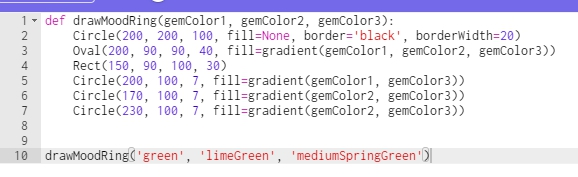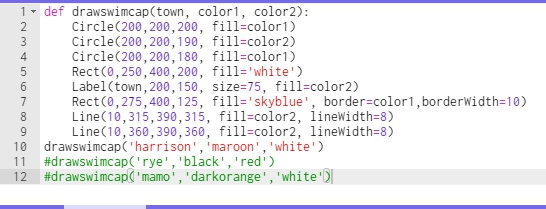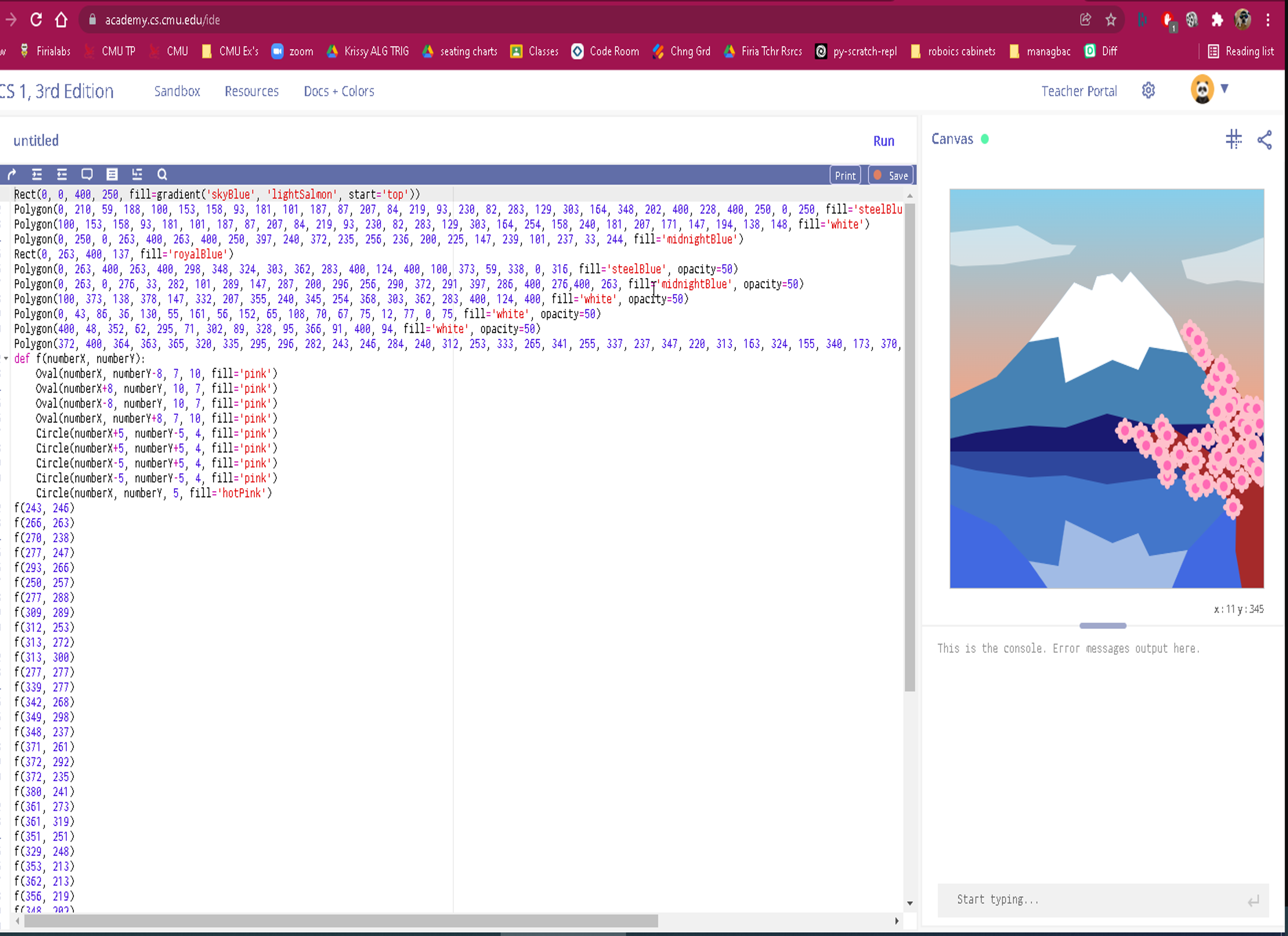# Array Fun 2 

Complete all the methods below. When you are done, copy and paste the testmethods.v4  into your class and run it to see if you have any obvious errors.

int sumEveryN(int[] nums, int n)

Description: This method returns the sum of every `n` elements of `num`s..

 Method Call return value/output sumEveryN( {1 , 2 , 3 , 4 }, 2 ) 4( ie 1 +3) sumEveryN( {13 , 42, 15, 33 , 44 , 16 , 52} ,3) 98 ( ie 13 + 33+ 52)

String[] doubleArr(String[] strs)

Description: This method returns a new version of `strs` in which each element now appears twice. This can be done with a for-each loop, which I believe is easier and more intuitive.

 Method Call return value/output doubleArr( {“a”,”b”,”c”} ) {“a”,”a”,”b”,”b”, “c” , “c”} doubleArr( {“math”,”ware”,”house”,”.com” }) {“math”,”math”,”ware”,”ware”,”house”,”house”,”.com”, “.com”}

int  indexOf5(int[] nums )

Description:This returns the index of the first occurrence element 5  or -1 if 5 does not appear anywhere in the array.

 Method Call return value/output indexOf5( { 2 , 3 , 5 , 4 } ) 2 indexOf5( { 2 , 3 , 5 , 4, 5  } ) 2 indexOf5( { 2 , 3 ,7  , 4, 3,   } ) -1

More Sample calls and return vals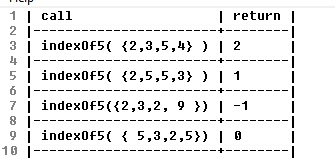int indexOf(int[] nums, int num)

Description: This method returns the index value of the first appearance of `num` or -1 if `num` is not an element of `nums` .

 Method Call return value/output indexOf( {6,4 ,7,3, 4 }, 4) 1 indexOf( {6,4 7 ,3,2,7}, 7) 2 indexOf( {6,4 ,2,3}, 22) -1

int[] randos(int start, int end, int howMany)

Description:  This method returns an array of random numbers between [start,end] . Note make sure that each element in the new array attempts to make a new random int.

More example calls and returns :

double meanBetween(int[] nums, int min, int max)

Description:  This method returns the mean of nums ; however, this method only counts values within the range (min,max) as shown in the examples below:

int secondSmallest(int[] nums )

Description: This method returns the element of `nums` with the second smallest value.

Note: You may not modify the input array. For instance, you may not put nums in order,  which would be bad because you were not asked to modify the array.

@precondition: nums.length >= 2

Note: You will lose credit if you use a constant to represent the smallest or second smallest number. See pseudocode

 Method Call return value/output secondSmallest( { 2 , 18 , 22, 4 , 6 } ) 4 secondSmallest( { 3 , 7 , 15 , 1 ,101} ) 3

The question below is extra credit .
** int[] add(int[] num1, int[] num2, int base) throws ArithmeticException
@precondition `1 < base < 11`
@precondition `num1.length = 5 and num2.length = 5`
@postcondition: the returned array has 5 elements representing the sum or an ArithmeticException is thrown

Description: This method attempts to replicate addition. Consider both num1 and num2 represent the five digits of a number. Each element in the array stores one of the digits. For instance, the number 143 would be represented as {0,0,1,4,3 }. Numbers can be in any base between 2 and 10 inclusive , and the number 101102 in binary would appear in an arrays as : {1, 0, 1 , 1, 0 } . Let’s assume the numbers are positive. You should return an array representing the digits of the sum of num1 and num2.  You may not do any math besides addition. You may not use any external libraries for any math or base conversions.   If the number of digits in the sum exceeds the maximum number of digits (5) , you should throw an arithmetic exception as shown in the code below:

Note: You may not use any kind of helper or utility methods that are built into pre-defined Java classes for converting numbers between bases. Only use techniques taught in this class.

 Method Call return value/output add( {0,0,0 ,4,2},{0,0,0,5,1}, 10) {0, 0, 0, 9, 3} ie (42 + 51 = 93) add( {0, 0, 0 ,7,2},{0,0,0,5,1}, 10) {0, 0 , 1 , 2, 3} ie (72 + 51 = 123) add( {0, 0 , 0 , 1 , 1}, { 0 , 0 , 0 , 1 }, 2) {0, 0 , 1 , 0 , 0} ie ( 112 + 12= 1002 )

Old versions:

Array Fun 1

Array F un 2 (v 1)

Array Fun 3 (resizing included)

# String 1 and Array 1 Exercises

Exercises for String 1 and Array 1 (no loops)

When you are done, you can copy and past the score() method below into your class. It will test some (but, by no means all) of the things that your code should do

Create a class called StrArr1 and add each method below into it.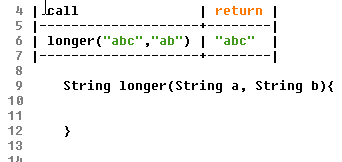For the absVals()  method below use the Math.abs() Java method.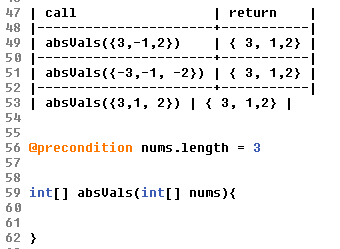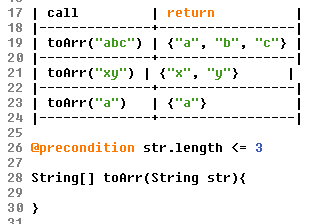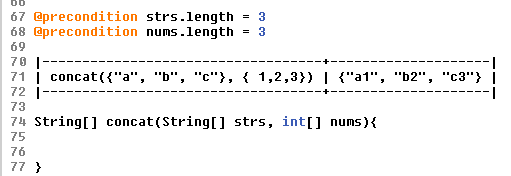For the  randos1to10()   method below use the  Math.random()  Java method. Create a new array to store random integers. Each integer should be [1,10]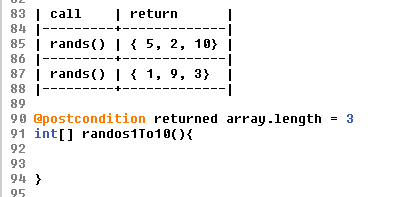# So, you want to Skip CS II

It is very rare that I recommend anyone skip from the Intro class, the easiest CS class,  to SUPA, the hardest CS class , without the foundation that we build in Computer Science II.

The path to skipping

If you  really want to try to skip CS II, the path would be to complete the online component of CS II curriculum during the school year and to then sit down with me for an interview. If I am satisfied that you are ready to skip CS II, I will then make the recommendation.

Much, though not all, of the CS II curriculum is online and so can be completed at a student’s convenience.

Please know, that most of the students who have followed this path and who found the Intro to CS class too easy and who then skipped into SUPA have ended up regretting this and, more often than not, they have had to drop the SUPA class.

Ask yourself– Would you skip Calculus I and instead jump right to Calculus II?

Probably not.

And it’s not much different here;  while it has been done,  for the most part it has only been successfully pulled off by students who are “hardcore” programmers and who have voluminous experience coding outside the classroom. Kids who have made their own apps and learned how to do real programming on their own.

Now, if that describes you and you have made real apps that you can show, then maybe this is the right move, but otherwise, this jump almost never is.

So, what students should do is this – Have your guidance counsellor sign you up for CS II for next year.   If you complete the online  part of the CS II curriculum, by June, then we can sit down and I will have an assessment of your readiness to make this radical jump.